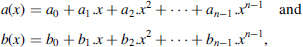## 8.3 OPERATIONS IN Zp[x]/f(x)

Given a polynomialof degree n (fn ≠ 0) whose coefficients belong to Zp (p prime), the set Zp[x] /f(x) of polynomials of degree less than n, modulo f(x), is a finite ring (Chapter 2, Section 2.2.2).

Given two polynomialsthe addition and the subtraction are defined as follows:where ai + bi and ai − bi are computed modulo p. Assume that two procedures

```procedure modular_addition (a, b: in coefficient; m: in
module; c: out coefficient);
procedure modular_subtraction (a, b: in coefficient; m: in
module; c: out coefficient);```

have been defined. They compute (a + b) mod m and (ab) mod m (see Sections 8.1.1 and 8.1.2). Then the addition and subtraction of polynomials are performed componentwise.

```for i in 0..n−1 loop
end loop;```

Algorithm 8.18 Subtraction of Polynomials

```for i in 0..n−1 loop
modular_subtraction (a(i), b(i), p, c(i));
end loop;```

### 8.3.2 Multiplication

Given two polynomials

their product z(x)=a(x).b(x) can be computed as follows:

The ...

Get Synthesis of Arithmetic Circuits: FPGA, ASIC and Embedded Systems now with the O’Reilly learning platform.

O’Reilly members experience books, live events, courses curated by job role, and more from O’Reilly and nearly 200 top publishers.